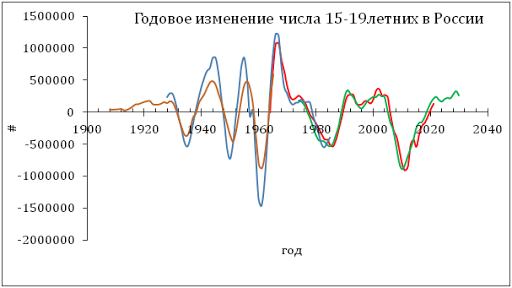## 7/13/11

### Okun's law integrated

In our previous post, we have estimated Okun’s law for the USA and several develop countries. This law links real economic growth and the change in unemployment rate. Here we integrate this relationship and obtain the dependence of the unemployment rate on real GDP. It allows modeling the rate of unemployment over time.

We have rewritten Okun’s law using the growth rate of real GDP per capita instead of GDP itself:

du = a + bdlnG (1)

where du is the annual increment in the rate of unemployment, dlnG=dG/G is the relative change rate of real GDP per capita per one year, a and b are empirical coefficients. Okun’s law suggests that b<0.

The reason to use per head values is obvious – the rate of unemployment is a population independent characteristic (i.e. normalized to total population) and GDP implicitly includes the change in population. When the change in population is fluctuating, relationship (1) is biased.

For the United States we have obtained the following relationship:

du = -0.42dlnG + 1.07, t<1985
du = -0.62dlnG + 1.09, t>1984 (2)

with a structural break in 1984. This was a preliminary assessment of the links as based on an eye-fit between measured and predicted curves. No formal minimization was applied.
When integrated between t0 and t, equation (1) can be rewritten in the following form:

u(t) = u(t0) + bln[G/G0] +a(t-t0) + c (3)

Without loss of generality, we assume t0=0. The intercept c≡0, as is clear for t=t0. Instead of integrating (3), we calculate cumulative sums of the annual estimates of du and lnG with appropriate initial conditions. The cumulative sum of du’s is the time series of the unemployment rate. Figure 1 depicts the measured and observed curves. The agreement is excellent and has been obtained by a formal statistical method.

We have re-estimates all coefficients in (3), and thus in (2), using a LSQ technique. Since we have already introduced a structural break in (2), we have sought the best fit allowing the year of this break to vary between 1970 and 1990. The best-fit (dynamic) model minimizing the RMS error of the cumulative model (3) is as follows:

du = -0.406dlnG + 1.113, t<1979
du = -0.465dlnG + 0.866, t>1978 (4)

This model suggests a smaller shift in the slope and a larger change in the intercept around 1979. The break year has changed from 1984 to 1979. This is a very important finding because both relationships in (4) give very close predictions for the period between 1978 and 1985. Therefore, the shift in coefficients in (4) is likely not an abrupt one but actually a transition process between two different states of the US economy.

The cumulative form of the dynamic Okun’s law (4) is characterized by standard error of 0.53% for the period between 1958 and 2010. The average rate of unemployment for the same period is 5.6% with an average annual increment of 1.06%. Figure 2 displays the cumulative model error.

Figure 1. The observed and predicted rate of unemployment in the USA between 1958 and 2010.
Figure 2. The residual error or the cumulative model (4).